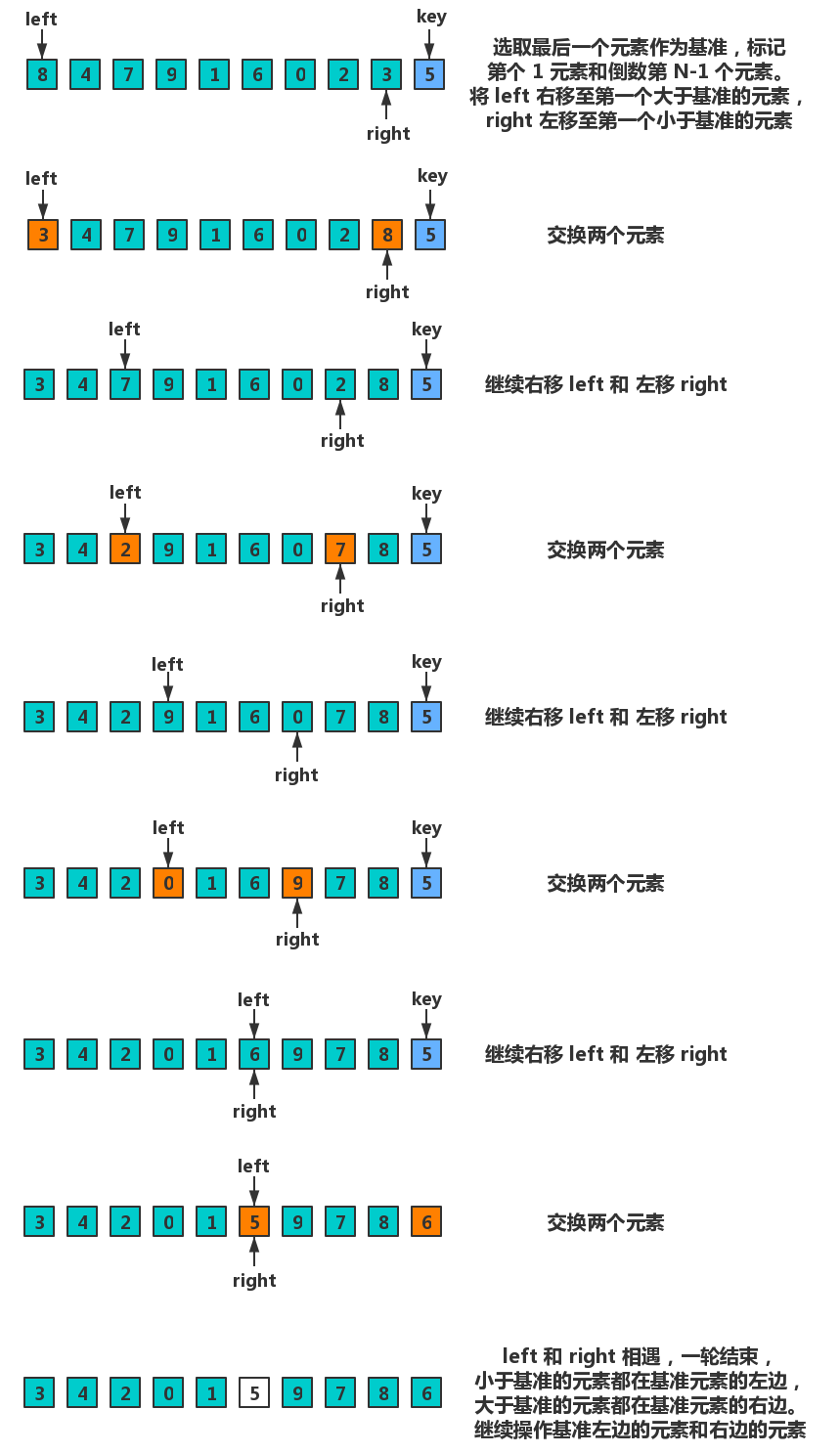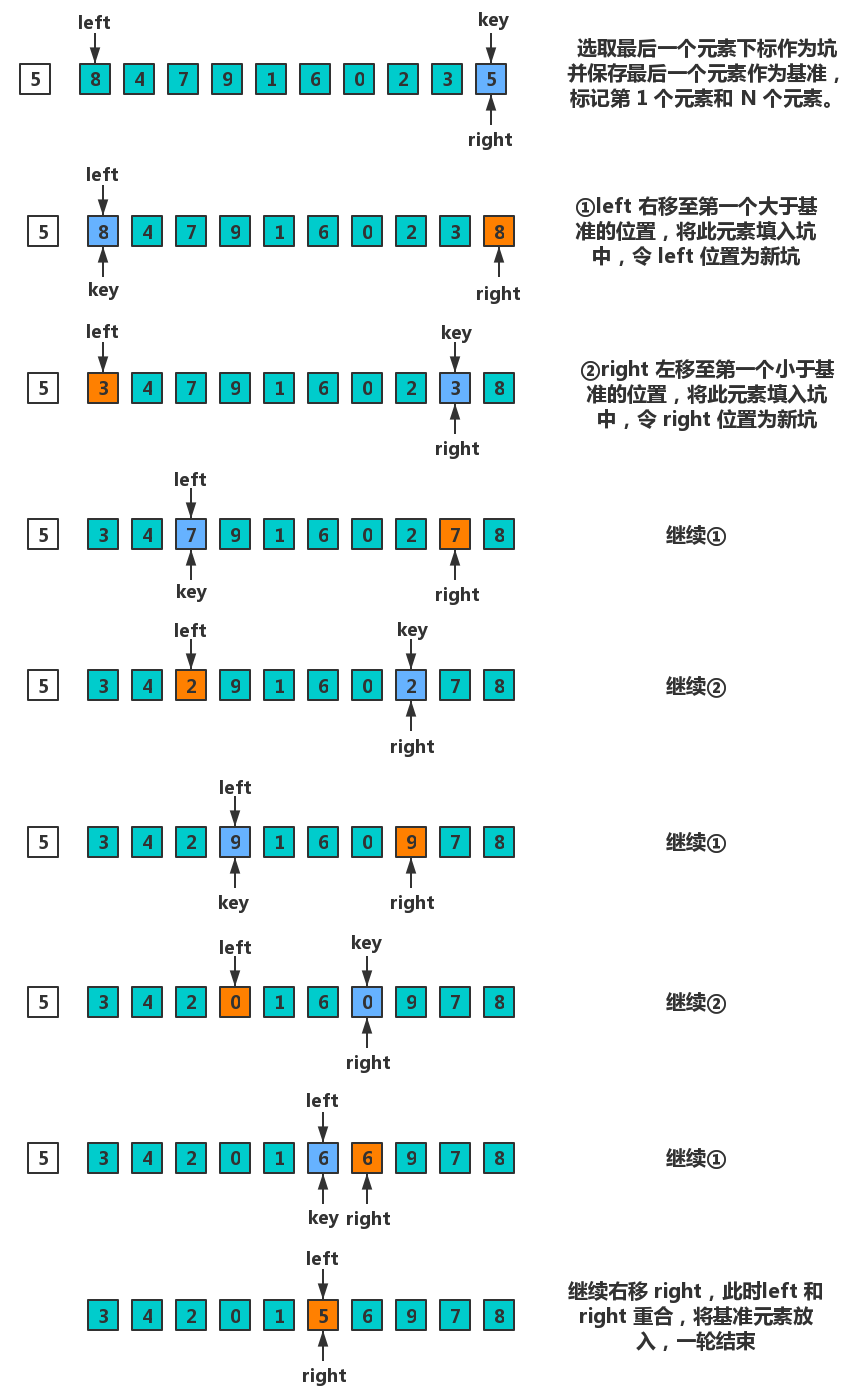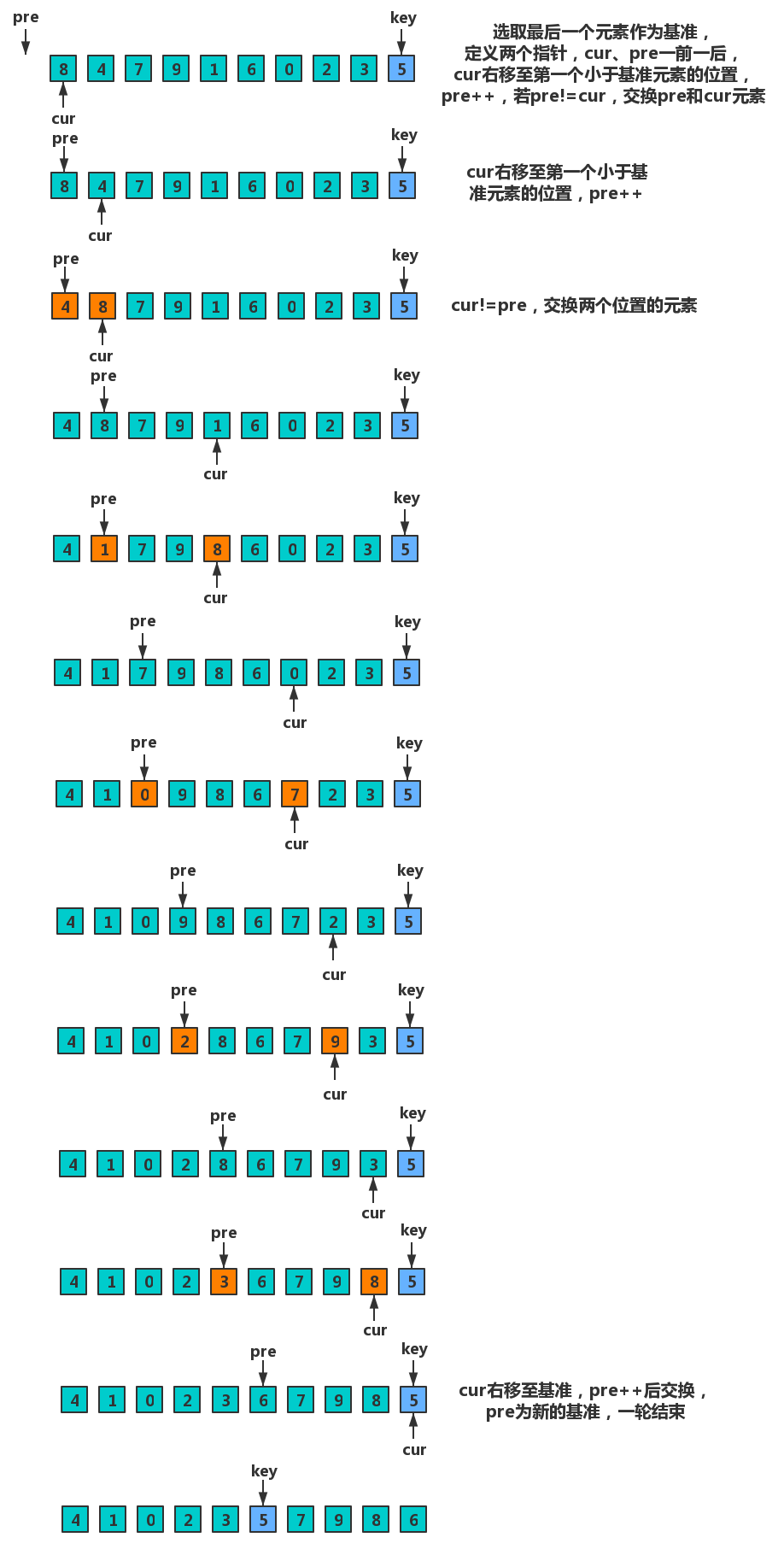# 具体实现

## 左右指针法

• 实现说明• 代码实现

``````  public static void sort1(int[] arr, int start, int end) {
if (start >= end) {
return;
}
int key = end;
int left = start;
int right = end - 1;
int temp;
//当left和right相遇时结束
while (left < right) {
//移动left至第一个大于基准的元素
while (left < right && arr[left] <= arr[key]) {
left++;
}
//移动right至第一个小于基准的元素
while (left < right && arr[right] >= arr[key]) {
right--;
}
//交换left和right元素
temp = arr[left];
arr[left] = arr[right];
arr[right] = temp;
}
//交换相遇位置和基准
if (arr[left] > arr[key]) {
temp = arr[left];
arr[left] = arr[key];
arr[key] = temp;
}
//递归
sort1(arr, start, left - 1);
sort1(arr, right + 1, end);
}``````
• 细节说明

1. 下面的代码为什么还要判断left < right？

`` while (left < right && arr[left] <= arr[key]) ``

key是序列的最后一个，right是key前一个位置，如果arr[right]=arr[key]，满足arr[left]<=arr[key]，然后left++，这时候left就会移动到key的位置

2. 出了while循环并不是直接进行交换

`````` if (arr[left] > arr[key]) {
temp = arr[left];
arr[left] = arr[key];
arr[key] = temp;
}``````

当left和right重合时，由于不满足left<right退出while循环不会继续右移left，若此时left元素依然小于基准元素则无需进行交换。

## 挖坑法

• 实现说明• 代码实现

``````  public static void sort2(int[] arr, int start, int end) {
if (start >= end) {
return;
}
int mark = arr[end];
int left = start;
int right = end;
int key = end;
//当left和right重合时结束
while (left < right) {
//left右移至第一个大于基准的位置
while (left < right && arr[left] <= mark) {
left++;
}
//将left元素填入坑中，令left位置为新坑
arr[key] = arr[left];
key = left;
//right左移至第一个小于基准的位置
while (left < right && arr[right] >= mark) {
right--;
}
//将right元素填入坑中，令right位置为新坑
arr[key] = arr[right];
key = right;
}
//将基准元素放入重合位置
arr[left] = mark;
sort2(arr, start, left - 1);
sort2(arr, right + 1, end);
}``````

由于坑位和right都为end，进行简化

``````  public static void sort3(int[] arr, int start, int end) {
if (start >= end) {
return;
}
int mark = arr[end];
int left = start;
int right = end;
//当left和right重合时结束
while (left < right) {
//left右移至第一个大于基准的位置
while (left < right && arr[left] <= mark) {
left++;
}
//将left元素填入坑中，令left位置为新坑
arr[right] = arr[left];
//right左移至第一个小于基准的位置
while (left < right && arr[right] >= mark) {
right--;
}
//将right元素填入坑中，令right位置为新坑
arr[left] = arr[right];
}
//将基准元素放入重合位置
arr[left] = mark;
sort2(arr, start, left - 1);
sort2(arr, right + 1, end);
}``````

## 前后指针法

• 实现说明• 代码实现

``````  public static void sort4(int[] arr, int start, int end){
if (start >= end) {
return;
}
int key = end;
int cur = start;
int pre = cur - 1;
int temp;
//结束循环时cur在基准处
while (cur < key) {
//当cur元素小于基准元素时才会pre++
if (arr[cur] < arr[key] && ++pre != cur) {
temp = arr[cur];
arr[cur] = arr[pre];
arr[pre] = temp;
}
cur++;
}
temp = arr[cur];
arr[cur] = arr[++pre];
arr[pre] = temp;
sort4(arr, start, pre - 1);
sort4(arr, pre + 1, end);
}``````

# 优化方案

## 相同元素相聚

1. 在划分过程中，把与key相等元素放入数组的两端

2. 划分结束后，把与key相等的元素移到基准周围